## Example Questions

← Previous 1

### Example Question #1 : How To Find The Solution To An Inequality With Division

Which of the following inequalities defines the solution set for the inequality 14 – 3x ≤ 5?

Possible Answers:

x ≤ 3

x ≤ –19/3

x ≥ 3

x ≥ –3

x ≤ –3

Correct answer:

x ≥ 3

Explanation:

To solve this inequality, you should first subtract 14 from both sides.

This leaves you with –3x ≤ –9.

In the next step, you divide both sides by –3, remembering to flip the inequality sign when you do this.

This leaves you with the solution x ≥ 3.

If you selected x ≤ 3, you probably forgot to flip the sign. If you selected one of the other solutions, you may have subtracted incorrectly.

### Example Question #2 : How To Find The Solution To An Inequality With Division

Solve 6x – 13 > 41

Possible Answers:

> 4.5

< 9

< 6

> 9

> 6

Correct answer:

> 9

Explanation:

Add 13 to both sides, giving you 6x > 54, divide both sides by 6, leaving > 9.

### Example Question #3 : How To Find The Solution To An Inequality With Division

Solve for.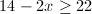Possible Answers: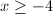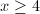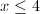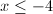Correct answer:Explanation: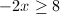When dividing both sides of an inequality by a negative number, you must change the direction of the inequality sign.### Example Question #4 : How To Find The Solution To An Inequality With Division

Solve 3 < 5x + 7

Possible Answers:

2 > x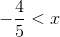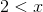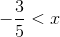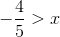Correct answer:Explanation:

Subtract seven from both sides, then divide both sides by 5, giving you –4/5 < x.

### Example Question #5 : How To Find The Solution To An Inequality With Division

Find is the solution set for x where: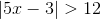Possible Answers: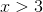or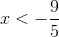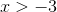or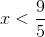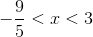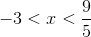Correct answer:orExplanation:

We start by splitting this into two inequalities,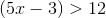and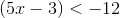We solve each one, giving usor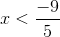.

### Example Question #41 : Inequalities

Which of the following could be a value of, given the following inequality?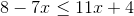Possible Answers: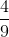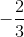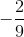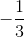Correct answer:Explanation:

The inequality that is presented in the problem is:Start by moving your variables to one side of the inequality and all other numbers to the other side: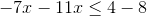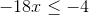Divide both sides of the equation by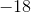. Remember to flip the direction of the inequality's sign since you are dividing by a negative number!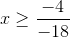Reduce: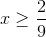The only answer choice with a value greater than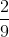is.

### Example Question #1 : Inequalities

Solve for the-intercept: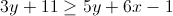Possible Answers:Correct answer:Explanation:

Don't forget to switch the inequality direction if you multiply or divide by a negative.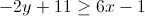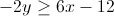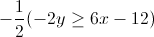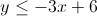Now that we have the equation in slope-intercept form, we can see that the y-intercept is 6.

### Example Question #3 : Inequalities

Solve for: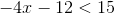Possible Answers: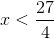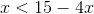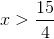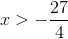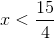Correct answer:Explanation:

Begin by addingto both sides, this will get the variable isolated: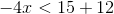Or...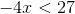Next, divide both sides by:Notice that when you divide by a negative number, you need to flip the inequality sign!

### Example Question #1 : Inequalities

Each of the following is equivalent to

xy/z * (5(x + y))  EXCEPT:

Possible Answers:

xy(5x + 5y)/z

5x²y + 5xy²/z

5x² + y²/z

xy(5y + 5x)/z

Correct answer:

5x² + y²/z

Explanation:

Choice a is equivalent because we can say that technically we are multiplying two fractions together: (xy)/z and (5(x + y))/1.  We multiply the numerators together and the denominators together and end up with xy (5x + 5y)/z.  xy (5y + 5x)/z is also equivalent because it is only simplifying what is inside the parentheses and switching the order- the commutative property tells us this is still the same expression.  5x²y + 5xy²/z is equivalent as it is just a simplified version when the numerators are multiplied out.  Choice 5x² + y²/z is not equivalent because it does not account for all the variables that were in the given expression and it does not use FOIL correctly.

### Example Question #1 : Inequalities

What is the solution set of the inequality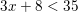?

Possible Answers: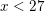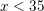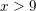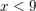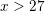Correct answer:Explanation:

We simplify this inequality similarly to how we would simplify an equation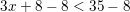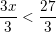Thus← Previous 1

### All ACT Math Resources